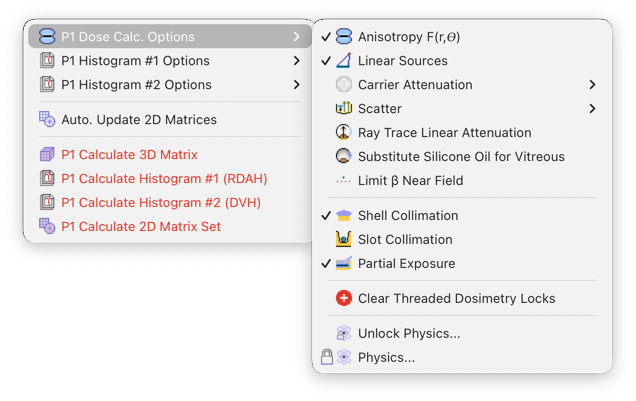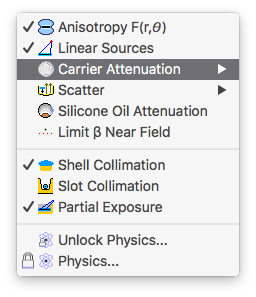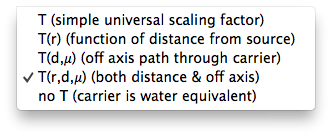Carrier

The carrier modifier attempts to correct for attenuation in the source carrier. This factor should be included in dose calculations if the density or atomic composition of the carrier differs significantly from water. An example of plaques that require this correction are the COMS plaques which use silastic carriers. Silastic is about 40% Si by weight and has an effective Z of about 10.7 compared to 7.4 for water. This factor should be disabled for plaques that do not use a carrier (eg BEBIG and USC plaques) or those that use acrylic carriers (eg ROPES plaques). Thus, for a COMS plaque that uses a silicone carrier, the carrier modifier should be enabled, and the scatter & flourescence factor B(r) factor should be disabled. For a COMS plaque that uses a gold insert instead of silicone, the carrier modifier should be disabled, and the scatter & flourescence factor B(r) factor should be enabled.

In earlier versions of Plaque Simulator, the modifier T was simply a scalar factor, typically about 0.9 for central axis distances out to about 1 cm for the COMS carriers, which was universally applied without regard to the location of the dose calculation point. This factor was based on measured data from Sou-Tung Chiu-Tsao et al, Dosimetry for I-125 seed (model 6711) in eye plaques, Medical Physics 20(2), 383-389, 1993 which includes scatter loss from the gold shell, so the Scatter Modifier B(r) is automatically turned off when the Carrier factor is enabled.

Plaque Simulator version 5 introduced T(r), T(μ) and T(r,μ) which are similar to T except that they vary as a function of the path length of primary radiation through the carrier and the distance r between the source and calculation point. The factor μ is considered to be an effective linear attenuation coefficient for the carrier material (roughly 0.1 per mm for silastic). A ray is cast from the center of each source towards the dose calculation point to estimate the average primary path length in the carrier. The factor T(μ) is an exponential function of the path length (less the radius of the source). T(r,μ) scales T(μ) by the ratio T(r)/T. You can choose which model for carrier correction to use from the Dose Calc. Options menu or by clicking on the Carrier button in the Rx window toolbar.When one of the T(...) items is checked in the Dose Calc. Options -> Carrier Attenuation menu, or the Carrier button in the Prescription windowis enabled, the carrier modifier T(r) is included in the dose calculation.